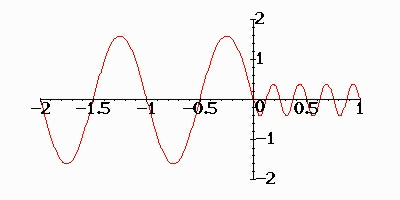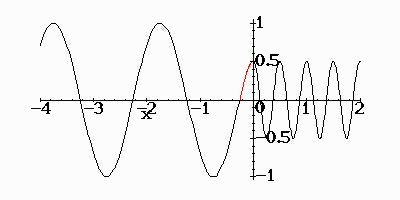### Impedance Matching Using Quarter Wavelength Medium

 Impedance Matching Using Quarter Wavelength MediumLight wave falling on a glass surface suffers reflection because of impedance mismatch. If Z_g = 250 Ohms (typical), the fraction of power reflection is[(Z_g - Z_0)/(Z_g + Z_0)]^2 = [(250-377)/(250+377)]^2 = 0.04 = 4%,where Z_0 = sqrt(mu_0/epsilon_0) = 377 Ohms is the impedance of free sapce.The first animation shows reflection and transmission of sinusoidal wave of unit amplitude at a boundary between Z_1 = 1 and Z_2 = 1/4. Because of reflection, (partial) standing waves are formed. Power transfer is evidently not very smooth.If a third medium having an impedance Z_3 = sqrt(Z_1*Z_2) (geometric mean of Z_1 and Z_2) and thickness of quarter wavelength (wavelength in the medium to be inserted) is inserted, wave reflection can be avoided entirely. The second animation shows this case. The red portion in the waveform corresponds to the inserted quarter wavelength medium. Note that there are no standing waves (no reflection) and all of the incident wave energy is transferred to the medium 2.Ċ
Jason Lin,
2018年9月6日 凌晨12:43